# Mensuration – Area of General Quadrilateral | Class 8 Maths

• Difficulty Level : Expert
• Last Updated : 26 Oct, 2020

General Quadrilateral can be defined as a closed two-dimensional shape that has four sides or edges, and also have four corners or vertices. In mensuration, the shape of objects are classified based on the number of sides of the quadrilateral. There are many types of quadrilateral all of them having four sides and the sum of angles of these shapes is 360 degrees.

As defined above any polygon having four sides is termed as a quadrilateral. Different types of quadrilateral are mentioned below:

• Rectangle
• Square
• Rhombus
• Parallelogram
• Trapezium
• Kite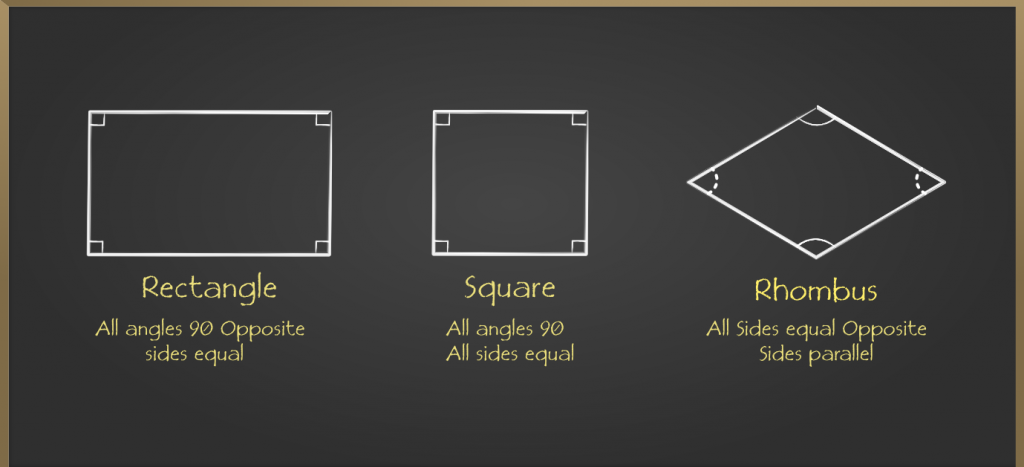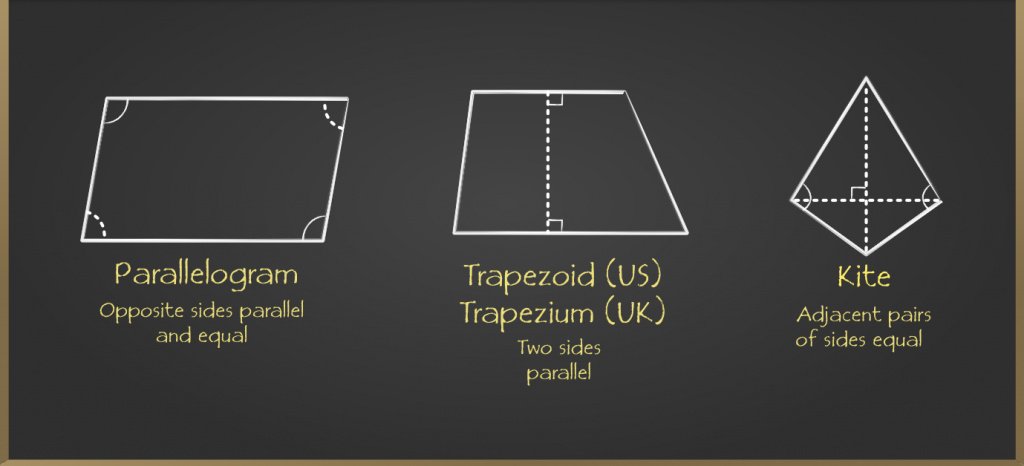• A closed figure having four sides.
• The summation of Interior angles of a quadrilateral is 360 degrees.
• The four sides can vary in length or maybe equal depending upon the type of quadrilateral.

### Area of a Rectangle

A rectangle is a closed figure having four sides in which opposite sides are equal and parallel to each other and the diagonals of the rectangles bisect at 90 degrees.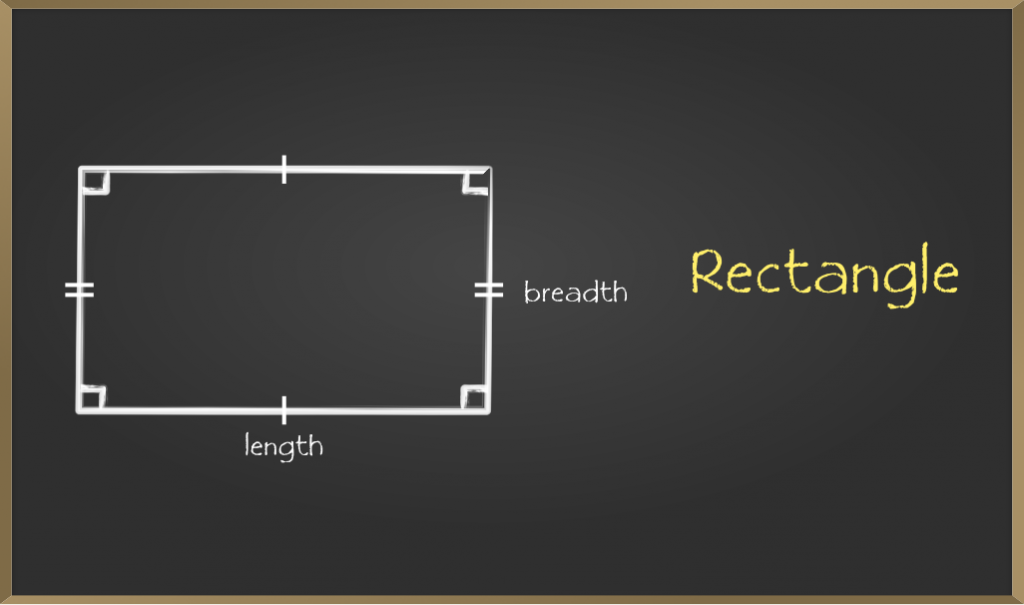Area of Rectangle = (Length x Breadth) meter square

Example: Given the length and breadth of a rectangle is 12 m and 6m. Calculate the area of the rectangle.

Solution: Since we know that,

Area of rectangle = Length x Breadth

= 12 x 6

= 72 meter square.

### Area of a Square

A square is a special case of a rectangle in which the four sides are equal and all the sides are parallel to each other. In a square diagonal bisect perpendicularly to each other.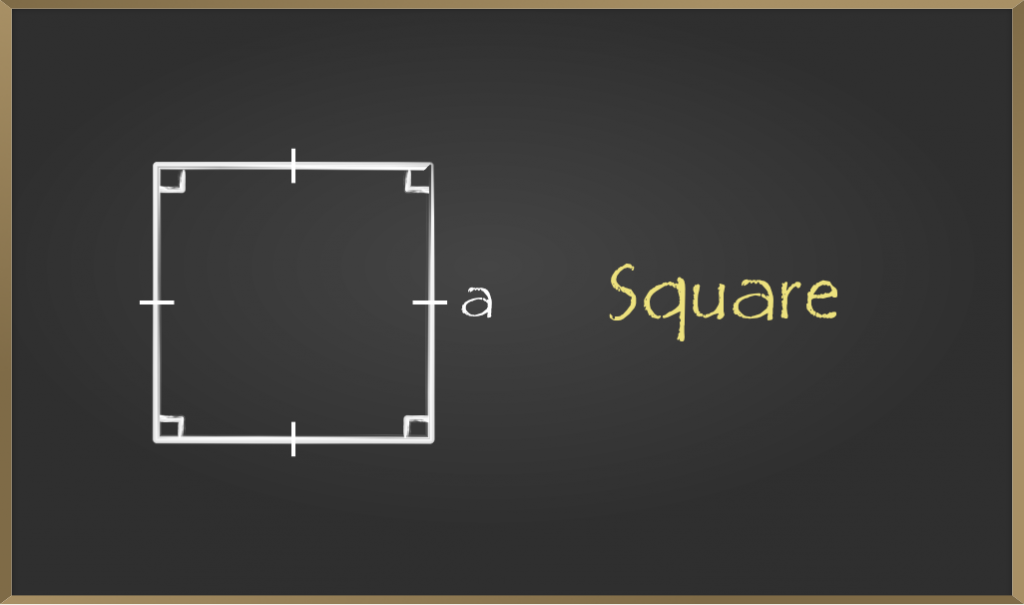Area of  Square =  (side x side ) meter square

Example: A quadrilateral having four sides each of length 7 m. Find the area .

Solution: we know the Area of Square= side x side (where side is the length)

= 7 x 7

= 49 meter square

### Area of Rhombus

A Rhombus is a special case of the square in which all the four sides and opposite angles are the same in measure and the opposite sides are parallel and the sum of the adjacent angles of a rhombus is equal to 180 degrees.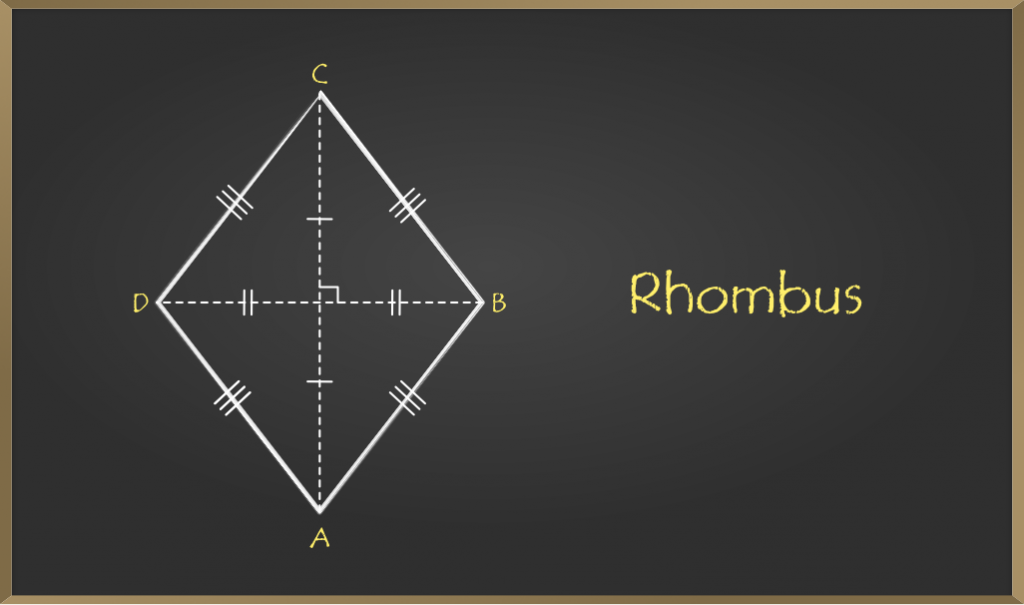Area of Rhombus = (1/2) x Diagonal 1 x Diagonal 2

Example: Given the area of the rhombus is 120-meter square then find the length of the one of the diagonal if the other diagonal is of length 12 m.

Solution: Since we know that,

Area of Rhombus = (1/2) x Diagonal 1 x Diagonal 2

Putting all the known values, we get-

120 = (1/2) x Diagoanl1 x 12

Diagonal2 = 20m

### Area of Parallelogram

The quadrilateral in which opposite sides are equal and parallel to each other are known as parallelograms. In this, diagonals bisect each other and the opposite angles are of equal measure in which the sum of two adjacent angles of a parallelogram is equal to 180 degrees.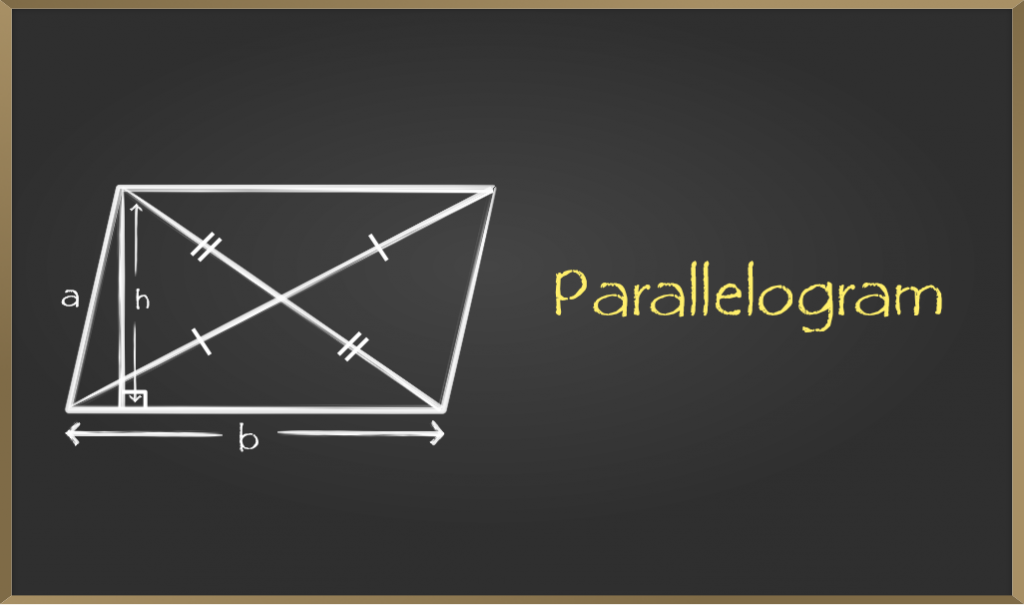Area of Parallelogram =(Base x Height ) meter square

Example: Calculate the area of a parallelogram, if the base and height are 10 and 15 m respectively.

Solution: Since we know that,

Area of Parallelogram = Base x Height

Putting all the known values

Area of Parallelogram = 10 x 15

Area of Parallelogram = 150 meter square

### Area of Trapezium

This quadrilateral is somewhat different from the others as there is only one pair of the opposite side of a trapezium is parallel to each other and the adjacent sides are supplementary to each other and the diagonals bisect each other in the same ratio.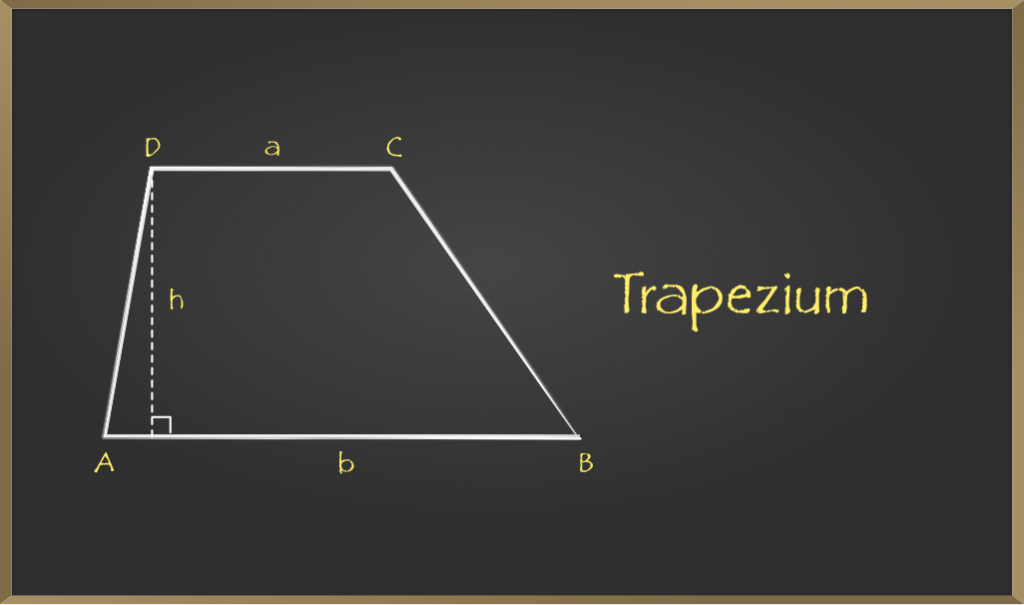Area of Trapezium = (1/2) h (AB+CD)

Example: Find the area of trapezium if height is 5 cm and AB and CD are given as 10 and 6 cm respectively.

Solution: Given, AB = 10cm

CD = 6cm

height = 5cm

According to the formulae,

Area of Trapezium = (1/2) h (AB+CD)

= 1/2 x 5 x 10 x 6

Area of Trapezium =150 cm cube

### Area of Kite

Kite is a special quadrilateral in which each pair of the consecutive sides is congruent, but the opposite sides are not congruent. In this, the largest diagonal of a kite bisect the smallest diagonal.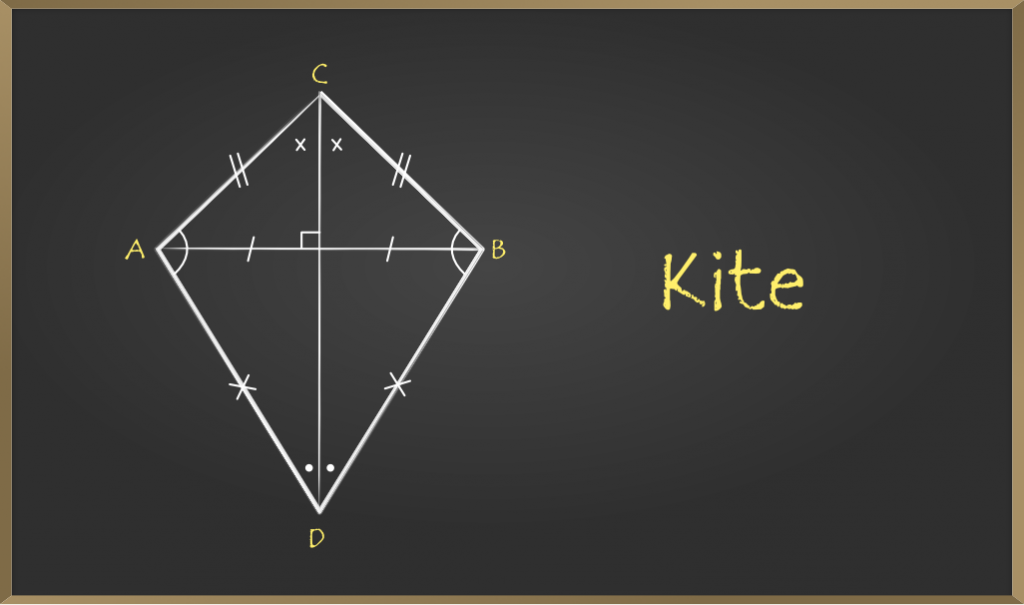Area of kite =1/2 x Diagonal 1 x Diagonal 2

where diagonal 1 = long diagonal of kite(KM)

diagonal 2 = short diagonal of kite(JL)

Example: Find the area of kite whose longest and shortest diagonals are 20cm and 10cm respectively.

Solution: Length of longest diagonal, D1= 20 cm

Length of shortest diagonal, D2= 10 cm

So,

Area of kite =1/2 x Diagonal 1 x Diagonal 2

Area of kite = 1/2 x 20 x 10 = 100cm2

My Personal Notes arrow_drop_up